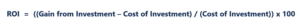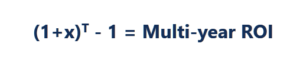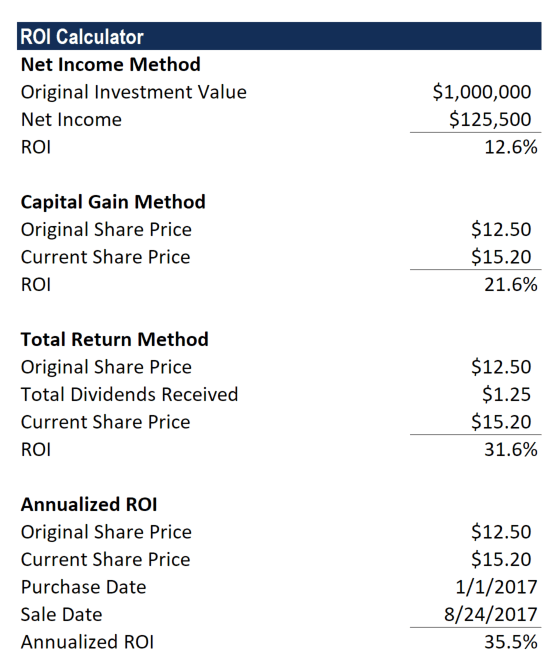# Return on Investment (ROI)

The benefit from investing in a certain resource

## What is Return on Investment (ROI)?

Return on Investment (ROI) is a performance measure used to evaluate the returns of an investment or to compare efficiency between different investments. ROI measures the return of an investment relative to the cost of the investment.

The Return on Investment (ROI) formula:Where “Gain from Investment” refers to the money generated from the sale of the investment, or the increase in value of the investment regardless of whether it is sold or not.### Breaking down Return on Investment

Return on Investment is a very popular financial metric due to the fact that it is a simple formula that can be used to assess the profitability of an investment. ROI is easy to calculate and can be applied to all kinds of investments.

Return on investment helps determine which investment opportunities are preferable to others.

For example, let us consider Investment A and Investment B, each with a cost of \$100. These two investments are risk-free (cash flows are guaranteed) and the cash flows are \$500 for Investment A and \$400 for Investment B next year.

Calculating the Return on Investment for both Investments A and B would give us an indication of which investment is better. In this case, the ROI for Investment A is (\$500-\$100)/(\$100) = 400%, and the ROI for Investment B is (\$400-\$100)/(\$100) = 300%. In this situation, Investment A would be the more favorable investment.

### Clearing Up Confusion: Return on Investment

1. Due to the fact that Return on Investment is expressed as a percentage (%) and not as a dollar amount, it can clear up confusion in dollar value returns.

For example, Investor A made \$200 investing in options and Investor B made \$50,000 investing in new condominiums. If only this information is given, you may assume that Investor B holds the better investment.

However, let us continue the example by assuming Investor A incurred costs of \$50 and Investor B incurred costs of \$40,000 to attain the respective \$200 and \$50,000 profits. These additional facts illustrate that the dollar value of return bears no significance without considering the cost of the investment. In this example, the return on investment for Investor A is (\$200-\$50)/(\$50) = 300% while the ROI for Investor B is (\$50,000-\$40,000)/(\$40,000) = 25%. Therefore, Investor A actually holds the better investment.

2. The time horizon must also be considered when you want to compare the ROI of two investments.

For example, Investment A has an ROI of 20% over a three-year time span while Investment B has an ROI of 10% over a one-year time span. If you were to compare these two investments, you must make sure the time horizon is the same. The multi-year investment must be adjusted to the same time horizon. To arrive at an average annual return, follow the steps below.

Changing a multi-year ROI into an annualized year formula:Where:

x = Annualized return

T = Time horizon

For Investment A with a return of 20% over a three-year time span, the annualized return is:

x = Annualized

T = 3 years

Therefore, (1+x)3 – 1 = 20%

Solving for x gives us an annualized ROI of 6.2659%. This is less than Investment B’s annual return of 10%.

To check if the annualized return is correct, assume the initial cost of an investment is \$20. After 3 years, \$20 x 1.062659 x 1.062659 x 1.062659 = \$24

ROI = (24 – 20) / (20) = 0.2 = 20%.

### Common Mistakes in Calculating ROI

ROI can be used for any type of investment. The only variation in investments that must be considered is how costs and profits are accounted for. Below are two examples how return on investment can be commonly miscalculated.

• Stocks: Investors commonly fail to incorporate transaction costs and dividend payouts into the ROI of stocks. Transaction costs are a cost to your investment while dividend payouts are a gain to your investment. The investor must take into account both the transaction cost and dividend gain to get an accurate return calculation. If this is not done, the ROI would be misrepresented.
• Real Estate: Investors commonly fail to incorporate rental income, taxes, insurance, and upkeep in the return on investment calculation of real estate. Rental income is a gain to your investment while taxes, insurance, and upkeep are costs to your investment.

It is important to account for the costs and gains of your investment throughout its entire lifespan, instead of merely taking the end of investment value and dividing it by initial cost.

### ROI Calculator in Excel

Download CFI’s free ROI Calculator in Excel to perform your own analysis.  The calculator uses the examples explained above and is designed so you can easily input your own numbers and see what the output is under different scenarios.

The calculator covers four different methods: net income, capital gain, total return, and annualized return.The best way to learn the difference between each of the four approaches is to input different numbers and scenarios and see what happens to the results.

### The Importance of ROI

Return on Investment, one of the most used profitability ratios, is a simple formula that measures the gain or loss from an investment relative to the cost of investment.

ROI is expressed as a percentage and is commonly used in making financial decisions, comparing companies’ profitability, and comparing the efficiency of different investments.

### Video Explanation of Return on Investment (ROI)

Watch this short video to quickly understand the main concepts covered in this guide, including the formula for calculating ROI and the reasons why ROI is a useful metric to look at.

CFI is a global provider of financial modeling courses and financial analyst certification. To learn more about evaluating investments, see the following additional resources:

### Other Resources

• Return on Assets
• Return on Equity
• Internal Rate of Return
• Investing: A Beginner’s Guide

### Financial Analyst Training

Get world-class financial training with CFI’s online certified financial analyst training program!

Gain the confidence you need to move up the ladder in a high powered corporate finance career path.

Learn financial modeling and valuation in Excel the easy way, with step-by-step training.